# NCERT Solutions For Class 9 Math Chapter – 8 Exercise – 8.2

1. ABCD is a quadrilateral in which P, Q, R and S are mid-points of the sides AB, BC, CD and DA (see Fig 8.29). AC is a diagonal. Show that:
(i) SR || AC and SR = 1/2 AC
(ii) PQ = SR
(iii) PQRS is a parallelogram.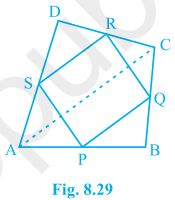Solution:

(i) In ΔDAC,

R is the mid point of DC and S is the mid point of DA.

Thus by mid point theorem, SR || AC and SR = ½ AC

(ii) In ΔBAC,

P is the mid point of AB and Q is the mid point of BC.

Thus

by mid point theorem, PQ || AC and PQ = ½ AC

also, SR = ½ AC

, PQ = SR

(iii) SR || AC ———————- from question (i)

and, PQ || AC ———————- from question (ii)

⇒ SR || PQ – from (i) and (ii)

also, PQ = SR

, PQRS is a parallelogram.

2. ABCD is a rhombus and P, Q, R and S are the mid-points of the sides AB, BC, CD and DA respectively. Show that the quadrilateral PQRS is a rectangle.

Solution: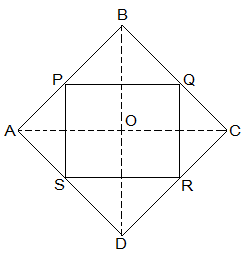Given in the question,

ABCD is a rhombus and P, Q, R and S are the mid-points of the sides AB, BC, CD and DA respectively.

To Prove,

PQRS is a rectangle.

Construction,

Join AC and BD.

Proof:

In ΔDRS and ΔBPQ,

DS = BQ (Halves of the opposite sides of the rhombus)

∠SDR = ∠QBP (Opposite angles of the rhombus)

DR = BP (Halves of the opposite sides of the rhombus)

, ΔDRS ≅ ΔBPQ [SAS congruency]

RS = PQ [CPCT]———————- (i)

In ΔQCR and ΔSAP,

RC = PA (Halves of the opposite sides of the rhombus)

∠RCQ = ∠PAS (Opposite angles of the rhombus)

CQ = AS (Halves of the opposite sides of the rhombus)

, ΔQCR ≅ ΔSAP [SAS congruency]

RQ = SP [CPCT]———————- (ii)

Now,

In ΔCDB,

R and Q are the mid points of CD and BC respectively.

⇒ QR || BD

also,

P and S are the mid points of AD and AB respectively.

⇒ PS || BD

⇒ QR || PS

, PQRS is a parallelogram.

also, ∠PQR = 90°

Now,

In PQRS,

RS = PQ and RQ = SP from (i) and (ii)

∠Q = 90°

, PQRS is a rectangle.

3. ABCD is a rectangle and P, Q, R and S are mid-points of the sides AB, BC, CD and DA respectively. Show that the quadrilateral PQRS is a rhombus.

Solution:Given in the question,

ABCD is a rectangle and P, Q, R and S are mid-points of the sides AB, BC, CD and DA respectively.

Construction,

Join AC and BD.

To Prove,

PQRS is a rhombus.

Proof:

In ΔABC

P and Q are the mid-points of AB and BC respectively

, PQ || AC and PQ = ½ AC (Midpoint theorem) — (i)

SR || AC and SR = ½ AC (Midpoint theorem) — (ii)

So, PQ || SR and PQ = SR

As in quadrilateral PQRS one pair of opposite sides is equal and parallel to each other, so, it is a parallelogram.

, PS || QR and PS = QR (Opposite sides of parallelogram) — (iii)

Now,

In ΔBCD,

Q and R are mid points of side BC and CD respectively.

, QR || BD and QR = ½ BD (Midpoint theorem) — (iv)

AC = BD (Diagonals of a rectangle are equal) — (v)

From equations (i), (ii), (iii), (iv) and (v),

PQ = QR = SR = PS

So, PQRS is a rhombus.

Hence Proved

4. ABCD is a trapezium in which AB || DC, BD is a diagonal and E is the mid-point of AD. A line is drawn through E parallel to AB intersecting BC at F (see Fig. 8.30). Show that F is the mid-point of BC.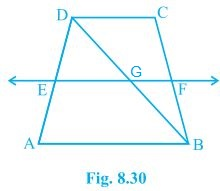Solution:

Given that,

ABCD is a trapezium in which AB || DC, BD is a diagonal and E is the mid-point of AD.

To prove,

F is the mid-point of BC.

Proof,

BD intersected EF at G.

E is the mid point of AD and also EG || AB.

Thus, G is the mid point of BD (Converse of mid point theorem)

Now,

In ΔBDC,

G is the mid point of BD and also GF || AB || DC.

Thus, F is the mid point of BC (Converse of mid point theorem)

5. In a parallelogram ABCD, E and F are the mid-points of sides AB and CD respectively (see Fig. 8.31). Show that the line segments AF and EC trisect the diagonal BD.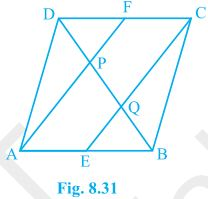Solution:

Given that,

ABCD is a parallelogram. E and F are the mid-points of sides AB and CD respectively.

To show,

AF and EC trisect the diagonal BD.

Proof,

ABCD is a parallelogram

, AB || CD

also, AE || FC

Now,

AB = CD (Opposite sides of parallelogram ABCD)

⇒½ AB = ½ CD

⇒ AE = FC (E and F are midpoints of side AB and CD)

AECF is a parallelogram (AE and CF are parallel and equal to each other)

AF || EC (Opposite sides of a parallelogram)

Now,

In ΔDQC,

F is mid point of side DC and FP || CQ (as AF || EC).

P is the mid-point of DQ (Converse of mid-point theorem)

⇒ DP = PQ — (i)

Similarly,

In ΔAPB,

E is midpoint of side AB and EQ || AP (as AF || EC).

Q is the mid-point of PB (Converse of mid-point theorem)

⇒ PQ = QB — (ii)

From equations (i) and (i),

DP = PQ = BQ

Hence, the line segments AF and EC trisect the diagonal BD.

Hence Proved.

6Show that the line segments joining the mid-points of the opposite sides of a quadrilateral bisect each other.

Solution: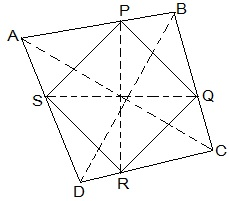Let ABCD be a quadrilateral and P, Q, R and S are the mid points of AB, BC, CD and DA respectively.

Now,

In ΔACD,

R and S are the mid points of CD and DA respectively.

, SR || AC.

Similarly we can show that,

PQ || AC,

PS || BD and

QR || BD

, PQRS is parallelogram.

PR and QS are the diagonals of the parallelogram PQRS. So, they will bisect each other.

7. ABC is a triangle right angled at C. A line through the mid-point M of hypotenuse AB and parallel to BC intersects AC at D. Show that
(i) D is the mid-point of AC
(ii) MD ⊥ AC
(iii) CM = MA = ½ AB

Solution: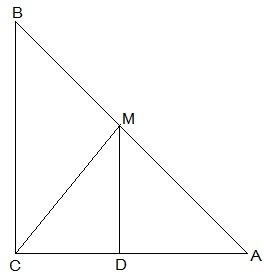(i) In ΔACB,

M is the midpoint of AB and MD || BC

, D is the midpoint of AC (Converse of mid point theorem)

(ii) ∠ACB = ∠ADM (Corresponding angles)

also, ∠ACB = 90°

, ∠ADM = 90° and MD ⊥ AC

(iii) In ΔAMD and ΔCMD,

AD = CD (D is the midpoint of side AC)